polybuffer

Create buffer around points, lines, or polyshape objects

Description

example

polyout = polybuffer(P,'points',d) returns a polyshape object with boundaries that buffer the 2-D points in P by a distance d. The polybuffer function computes the buffer by taking the union of circles of radius d centered at each point in P. The first column of the matrix P contains the x-coordinates of the points to buffer and the second column contains the corresponding y-coordinates.

Once you create the polyshape object polyout, you can analyze its properties or perform additional computations using polyshape functions. For example, you can access the vertices that define the buffered shape with the property polyout.Vertices, and you can plot the shape using the command plot(polyout).

example

polyout = polybuffer(P,'lines',d) returns a polyshape object with boundaries that buffer the line segments defined by the 2-D points in P. The polybuffer function computes the buffer by moving a circle of radius d along the line segments created by the input points, centered on the line segments.

example

polyout = polybuffer(P,'lines',d,'JointType',jt) specifies how the meeting points of line segment endpoints (joints) are treated. For example, polybuffer(P,'lines',d,'JointType','square') squares off the joints.

polyout = polybuffer(P,'lines',d,'JointType','miter','MiterLimit',lim) specifies a miter limit when the joint type is specified as 'miter'. The miter limit is the maximum allowable ratio between the distance a joint vertex is moved and the buffer distance d. The limit lim must be greater than or equal to 2.

example

polyout = polybuffer(polyin,d) returns a polyshape object with boundaries that buffer the input polyshape object polyin by a distance d. For positive values of d, solid region boundaries of polyin expand by d units, and hole boundaries shrink by d units. Negative values of d shrink solid boundaries and expand hole boundaries.

example

polyout = polybuffer(polyin,d,'JointType',jt) specifies how the joints of the polyshape are treated when the first input argument is a polyshape.

polyout = polybuffer(polyin,d,'JointType','miter','MiterLimit',lim) specifies a miter limit when the joint type is specified as 'miter' and the first input argument is a polyshape.

Examples

collapse all

Create a matrix that contains the 2-D coordinates of three points. Then, create a polyshape object that buffers each point by a distance of 0.25 units.

P = [0 0; 1 1; 2 1];
polyout = polybuffer(P,'points',0.25)
polyout =
polyshape with properties:

Vertices: [542x2 double]
NumRegions: 3
NumHoles: 0

Plot the original points and their buffer regions.

plot(P(:,1),P(:,2),'r.','MarkerSize',10)
hold on
plot(polyout)
axis equal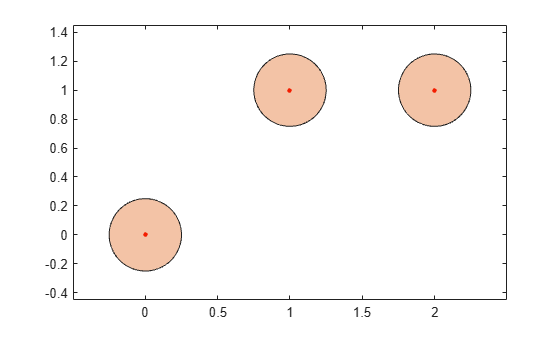Create buffer regions surrounding line segments.

Create a matrix of 2-D points, and compute a polyshape object that buffers the line segments connecting the points.

P = [0 0; 1 1; 2 1];
polyout1 = polybuffer(P,'lines',0.25);
plot(P(:,1),P(:,2),'r.','MarkerSize',10)
hold on
plot(polyout1)
axis equal
hold off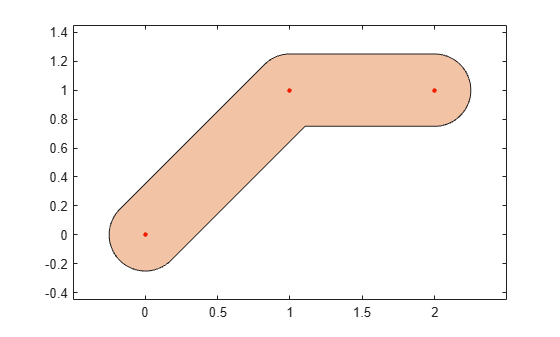By default, polybuffer rounds out the joints that connect line segments. You can control the shape of joints by using the 'JointType' name-value pair. For example, use the value 'miter' to preserve the angle connecting the two line segments.

figure
polyout2 = polybuffer(P,'lines',0.25,'JointType','miter');
plot(P(:,1),P(:,2),'r.','MarkerSize',10)
hold on
plot(polyout2)
axis equal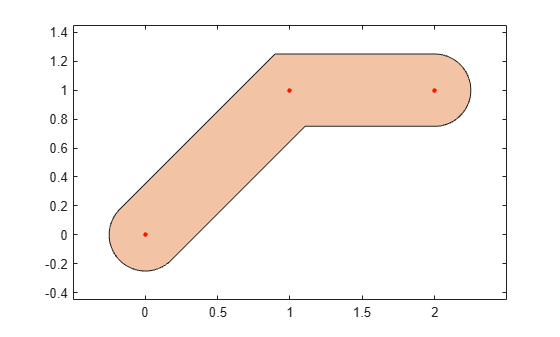Create a polygon with a solid boundary and a hole boundary. Then, create a buffer at a distance of 0.1 from the boundaries. By default the buffer has rounded joints.

polyin = polyshape({[0 0 1 3],[0.5 1.5 1.5 0.5]},{[0 3 3 0],[0.5 0.5 1.5 1.5]});
plot(polyin)
polyout1 = polybuffer(polyin,0.1);
hold on
plot(polyout1)
hold off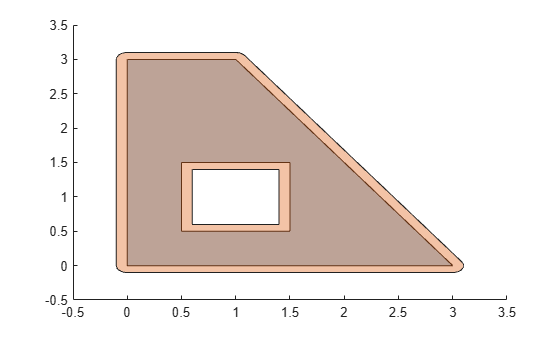Create a buffer using a miter limit of 2. The miter limit controls the pointiness of the joints.

polyout2 = polybuffer(polyin,0.1,'JointType','miter','MiterLimit',2);
plot(polyin)
hold on
plot(polyout2)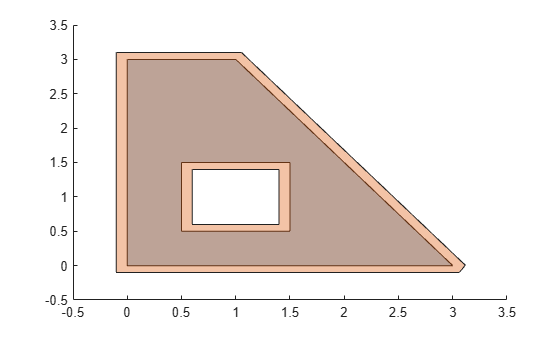Input Arguments

collapse all

Input vertices of 2-D points, specified as a 2-column numeric matrix. The first column of P contains the x-coordinates and the second column contains the corresponding y-coordinates.

Input polyshape, specified as a scalar, vector, matrix, or multidimensional array. When polyin is an array of polyshape objects, polybuffer applies the specified buffer parameters to each element.

Buffer distance, specified as a numeric scalar.

When the first input argument is a set of vertices, d must be a positive numeric scalar.

When the first input argument is a polyshape, d can be positive, negative, or zero:

• If d>0, then solid boundaries grow by a distance d and hole boundaries shrink.

• If d<0, then solid boundaries shrink by a distance d and hole boundaries grow.

• If d=0, then there is no change to the input boundaries.

Joint type for buffer boundaries, specified as one of the following:

• 'round' — Round out boundary corners.

• 'square' — Square off boundary corners.

• 'miter' — Limit the ratio between the distance a joint vertex is moved and the buffer distance to 3. This limit prevents excessive pointiness.

When the first input argument is a set of vertices, polybuffer applies the joint type only where endpoints of two line segments meet, and not at an open endpoint of a line segment.

When the first input argument is a polyshape, polybuffer only applies the joint type to solid boundaries when the buffer distance is positive, or for hole boundaries when the buffer distance is negative.

Miter limit, specified as a positive numeric scalar greater than or equal to 2. The miter limit is the ratio between the distance a joint vertex is moved and the buffer distance. Setting a miter limit controls the pointiness of boundary joints.